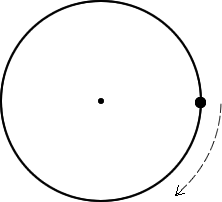This assignment is to be completed and turned in before taking the mastery test. When it is turned in, I will check that it is complete. You will take it and check solutions in the blue answer book. Every problem must be completed. Get help from me or another student if you are having trouble!
 On the circle below, draw the tangential velocity vector.On the circle below, draw the radius vector.On the circle below, draw the position vector.On the circle below, draw the centripetal force vector.On the circle below, draw the centripetal acceleration vector.Every question on this assignment refers to uniform circular motion. (Speed does not change.)

(1) A spinning toy top rotates 200 times in one minute. The top has a diameter of 5 cm.

(a) What is the period of motion for the top?
(b) What is the frequency of the top?
(c) What is the tangential velocity of the top at its edge?

(2) At an amusement park. Passengers ride in a special swing set where they are flung around in a horizontal circle. The radius is 45 m and it takes 1 minute to go around twice.

(a) What is the centripetal acceleration of the riders in the swings?
(b) What is the tangential velocity of the riders?
(c) This ride is made up of two circles of riders. The top of this ride spins as one piece. The riders on the inside have a radius that is 3/5 the radius of the riders on the outside. By what factor does the outside riders’ velocity compare to the inside riders’ velocity. (Hint: Think about how the period of motion for the two riders compares.)
(d) What centripetal force acts on a 50 kg rider?(Image credit: Margaritka: website: http://www.flickr.com/photos/88427556@N00/293572686/

(3) A racecar driver experiences a centripetal acceleration of 5 g’s while traveling around a horizontal curve with a speed of 75 m/s. What is the radius of the curve?
(4) On a different racetrack, a racecar driver travels from the inside of the curve to the outside of a horizontal curve. The radius changes by a factor of 7/8 and the centripetal acceleration changes by a factor of 3/4. By what factor would their new velocity compare to the old velocity?

(5) A centrifuge is used to separate plasma from blood by spinning very fast. A centrifuge has a radius of 22 cm. The sample in the centrifuge spins with an acceleration of 8568 m/s2.

(a) If a sample has a mass of 0.023 kg, then what centripetal force does is exert on it?
(b) What is the tangential velocity of this centrifuge in miles per hour?Image Credit: KEMIJSKO - TEHNOLOŠKI
FAKULTE,website:http://www.ktf-split.hr/glossary/image/centrifuge.gif

(6) A ride at the Dogwood festival spins the rider in a horizontal circle with a centripetal acceleration of 1.5 g’s. The ride’s radius is 5 meters.

(a) How much time does it take to rotate once?
(b) What is the rider’s tangential velocity?

(7) How would the new centripetal force compare to the old if the mss remained unchanged while the radius was halved and the doubled velocity?

by Tony Wayne ...(If you are a teacher, please feel free to use these resources in your teaching.)